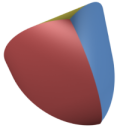The Reuleaux Triangle Tetrahedron:The Reuleaux TriangleReuleaux Triangle The Reuleaux triangle is the intersection of three circles of radius s centered at the vertices of an equilateral with side length s. It is named after Franz Reuleaux who was the principal of the Technische Hochschule Charlottenburg in 1890, the very school that today is the Technical University of Berlin. The Reuleaux triangle is the simplest nontrivial example of a curve of constant width - a curve in which the distance between two opposite parallel tangent lines to its boundary is the same, regardless of the direction of those two parallel lines. The Reuleaux TetrahedronReuleaux Tetrahedron The name Reuleaux Tetrahedron refers to the intersection of four spheres of radius s centered at the vertices of a regular tetrahedron with side length s. The sphere through each vertex passes through the other three vertices, which also form vertices of the Reuleaux tetrahedron. The Reuleaux tetrahedron has the same face structure as a regular tetrahedron, but with curved faces: four vertices, and four curved faces, connected by six circular-arc edges. In contrast to the Reuleaux Triangle it does not have constant width. The Reuleaux Triangle TetrahedronReuleaux Triangle Tetrahedron Our tetrahedron is constructed differently but nevertheless deserves the name Reuleaux. It is built from four Reuleaux triangles which are identified along their sides. Thus it can be made from paper. We constructed it as a polyhedron using the Alexandrov Polyhedron Editor. The Applet shows the polyhedron with colored Reuleaux Triangles. Use the left mouse button to rotate the object. The "e" key centers the tetrahedron.Click to view the applet The applet was written by Stefan Sechelmann a member of the Research Group Polyhedral Surfaces. For the general construction method see A. I. Bobenko, I. Izmestiev. Alexandrov's theorem, weighted Delaunay triangulations, and mixed volumes. Preprint arXiv:math.DG/0609447, 2006. Stefan Sechelmann 11-15-2006